# 5-demicube facts for kids

Kids Encyclopedia Facts

Demipenteract
(5-demicube)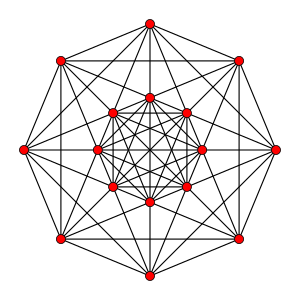Petrie polygon projection
Type Uniform 5-polytope
Family (Dn) 5-demicube
Families (En) k21 polytope
1k2 polytope
Coxeter
symbol
121
Schläfli
symbols
{3,32,1} = h{4,33}
s{2,4,3,3} or h{2}h{4,3,3}
sr{2,2,4,3} or h{2}h{2}h{4,3}
h{2}h{2}h{2}h{4}
s{21,1,1,1} or h{2}h{2}h{2}s{2}
Coxeter
diagrams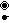=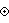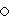4-faces 26 10 {31,1,1}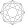16 {3,3,3}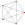Cells 120 40 {31,0,1}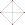80 {3,3}Faces 160 {3}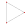Edges 80
Vertices 16
Vertex
figure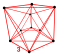rectified 5-cell
Petrie
polygon
Octagon
Symmetry D5, [32,1,1] = [1+,4,33]
+
Properties convex

In five-dimensional geometry, a demipenteract or 5-demicube is a semiregular 5-polytope, constructed from a 5-hypercube (penteract) with alternated vertices removed.

It was discovered by Thorold Gosset. Since it was the only semiregular 5-polytope (made of more than one type of regular facets), he called it a 5-ic semi-regular. E. L. Elte identified it in 1912 as a semiregular polytope, labeling it as HM5 for a 5-dimensional half measure polytope.

Coxeter named this polytope as 121 from its Coxeter diagram, which has branches of length 2, 1 and 1 with a ringed node on one of the short branches,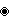and Schläfli symbol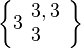$\left\{3 \begin{array}{l}3, 3\\3\end{array}\right\}$ or {3,32,1}.

It exists in the k21 polytope family as 121 with the Gosset polytopes: 221, 321, and 421.

The graph formed by the vertices and edges of the demipenteract is sometimes called the Clebsch graph, though that name sometimes refers to the folded cube graph of order five instead.

Family An Bn I2(p) / Dn E6 / E7 / E8 / F4 / G2 Hn
Regular polygon Triangle Square p-gon Hexagon Pentagon
Uniform polyhedron Tetrahedron OctahedronCube Demicube Dodecahedron • Icosahedron
Uniform 4-polytope 5-cell 16-cellTesseract Demitesseract 24-cell 120-cell • 600-cell
Uniform 5-polytope 5-simplex 5-orthoplex • 5-cube 5-demicube
Uniform 6-polytope 6-simplex 6-orthoplex • 6-cube 6-demicube 122 • 221
Uniform 7-polytope 7-simplex 7-orthoplex • 7-cube 7-demicube 132 • 231 • 321
Uniform 8-polytope 8-simplex 8-orthoplex • 8-cube 8-demicube 142 • 241 • 421
Uniform 9-polytope 9-simplex 9-orthoplex • 9-cube 9-demicube
Uniform 10-polytope 10-simplex 10-orthoplex • 10-cube 10-demicube
Uniform n-polytope n-simplex n-orthoplex • n-cube n-demicube 1k2 • 2k1 • k21 n-pentagonal polytope
Topics: Polytope families • Regular polytope • List of regular polytopes and compounds5-demicube Facts for Kids. Kiddle Encyclopedia.